Math Is Fun Forum

Discussion about math, puzzles, games and fun.   Useful symbols: ÷ × ½ √ ∞ ≠ ≤ ≥ ≈ ⇒ ± ∈ Δ θ ∴ ∑ ∫  π  -¹ ² ³ °

You are not logged in.

#851 2007-10-23 22:42:18

Maelwys
Member
Registered: 2007-02-02
Posts: 161

Re: 0.9999....(recurring) = 1?

Thanks for your concern for my love life. I considered finding a girlfriend, but then decided that if I did it wouldn't be fair to my wife and son. Especially since my wife is pregnant with our second child right now. I've heard that you're not supposed to get a girlfriend once you're married with a couple kids... something about "cheating".

;-)

Offline

#852 2007-10-23 22:49:31

Identity
Member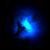Registered: 2007-04-18
Posts: 934

Re: 0.9999....(recurring) = 1?

Anthony I have another question for you. What do you think the following infinite sum is equal to?

Offline

#853 2007-10-23 22:52:40

MathsIsFunRegistered: 2005-01-21
Posts: 7,710

Re: 0.9999....(recurring) = 1?

Anthony ... stop being rude or I will have to ban you.

"The physicists defer only to mathematicians, and the mathematicians defer only to God ..."  - Leon M. Lederman

Offline

#854 2007-10-23 23:36:48

Identity
MemberRegistered: 2007-04-18
Posts: 934

Re: 0.9999....(recurring) = 1?

Anthony.R.Brown wrote:

and doing something far more interesting with their Life!...

So you think maths is uninteresting?

Offline

#855 2007-10-24 00:14:12

Dross
Member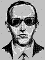Registered: 2006-08-24
Posts: 325

Re: 0.9999....(recurring) = 1?

This is taking a more personal tone because people are getting frustrated. Let's not allow it to degrade into an insult-hurling contest, though!

Offline

#856 2007-10-24 02:34:15

Anthony.R.Brown
Banned
Registered: 2006-11-16
Posts: 516

Re: 0.9999....(recurring) = 1?

Let´s Answer these  one at a Time!...

Quote: " Thanks for your concern for my love life. I considered finding a girlfriend, but then decided that if I did it wouldn't be fair to my wife and son. Especially since my wife is pregnant with our second child right now. I've heard that you're not supposed to get a girlfriend once you're married with a couple kids... something about "cheating". "

A.R.B

You have as much chance of having a Wife! as me Winning the Lottery!...

--------------------------------------------------------------------------------------------------------------------------------------------------------

Quote: " Anthony I have another question for you. What do you think the following infinite sum is equal to?  "

A.R.B

What ever they equal! the Fractions! have nothing to do with the Decimal Problem Infinite/Recurring 0.9

-------------------------------------------------------------------------------------------------------------------------------------------------------

Quote:" Anthony ... stop being rude or I will have to ban you. "

A.R.B

HAHAHAHAHAHAHAHAHAHAHA!!!!!!!!

--------------------------------------------------------------------------------------------------------------------------------------------------------

Quote: " and doing something far more interesting with their Life!...
So you think maths is uninteresting? "

A.R.B

In no way is it uninteresting? Just certain People make it that Way!...

--------------------------------------------------------------------------------------------------------------------------------------------------------

Quote:" This is taking a more personal tone because people are getting frustrated. Let's not allow it to degrade into an insult-hurling contest, though! "

A.R.B

No Let´s Not!!...Including Moderators!...

Last edited by Anthony.R.Brown (2007-10-24 03:08:33)

Offline

#857 2007-10-24 07:41:38

Devantè
Real Member
Registered: 2006-07-14
Posts: 6,400

Re: 0.9999....(recurring) = 1?

Anthony.R.Brown, this is a serious matter. MathsIsFun is not one to be mocked. Being the absolute creator of Maths Is Fun and the forum itself, without him you wouldn't even have anything to post on. To argue your point (which you seem to enjoy).

Now let´s get back to the serous people that come on this site! that are prepared to talk Math! and argue their case! Rather than coming on for five minutes! making ill informed and derogatory remarks! then run away with their tail between their legs! because they have no substance for what they believe in!...

Bah. Stop saying that. It's making you look as if you contradict every statement you say.

Insulting someone personally takes the argument to another level. Perhaps when you have been enlightened enough, you will look back on your posts and see what you have created here.

Offline

#858 2007-10-24 09:22:41

MathsIsFunRegistered: 2005-01-21
Posts: 7,710

Re: 0.9999....(recurring) = 1?

Anthony ... you were rude again.

You are temporarily banned.

"The physicists defer only to mathematicians, and the mathematicians defer only to God ..."  - Leon M. Lederman

Offline

#859 2007-10-28 16:08:11

mathsguenis
Member
Registered: 2007-10-28
Posts: 2

Re: 0.9999....(recurring) = 1?

hey i dont get it eatha i have spent hours and hours working on this and reading messages explaing this and still dont get it so ur not alone ...........................:cool:

Offline

#860 2007-11-01 19:37:51

landof+
Member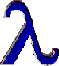Registered: 2007-03-24
Posts: 131

Re: 0.9999....(recurring) = 1?

they just don't equal... the main thing to remember is that any multiple of 3 cannot be a factor of 10^1, 10^2, 10^3, 10^4 and so on.

I shall be on leave until I say so...

Offline

#861 2007-11-01 19:55:57

Identity
MemberRegistered: 2007-04-18
Posts: 934

Re: 0.9999....(recurring) = 1?

landof+ wrote:

they just don't equal... the main thing to remember is that any multiple of 3 cannot be a factor of 10^1, 10^2, 10^3, 10^4 and so on.

Gee, that's an interesting thought... you would think that 0.999... is divisible by 3, but unfortunately you can only talk about divisibility when you are working with integers. Divisibility doesn't play much of a role in the 0.999... = 1 argument.

Last edited by Identity (2007-11-01 22:02:34)

Offline

#862 2007-11-01 21:56:08

Khushboo
Member
Registered: 2007-10-16
Posts: 47

Re: 0.9999....(recurring) = 1?

0.99999....=0.9+0.09+0.009+0.0009+0.00009+0.000009......infinity

This can be arranged in geometric series with sum to infinity

The sum to infinity formula can be used=> Sum=a/1-r  also(-1<r<1)

where a is the first term and r is the common ratio

Sum=0.9/1-0.1
=0.9/0.9
= 1
or 0.9999.....=1

Offline

#863 2007-11-01 22:56:46

Khushboo
Member
Registered: 2007-10-16
Posts: 47

Re: 0.9999....(recurring) = 1?

When i was trying to answer this question i didnt realise that this topic was posted way back. I was never able to see the last post ....it was later that i found out my insight to this question was no good as people have posted fantastic results for this question.....

regards
khushboo

Offline

#864 2007-11-05 15:01:03

mason777
Member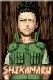Registered: 2007-11-05
Posts: 44

Re: 0.9999....(recurring) = 1?

Zach wrote:

I've done that with 0.27 recurring. It's quite a logical thought.Thats funny know what i learned?
When life gives you lemons squirt lemon juice in the eyes of your enemies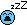>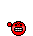Man, what a pain...

Offline

#865 2007-11-05 23:13:37

JohnnyReinB
MemberRegistered: 2007-10-08
Posts: 453

Re: 0.9999....(recurring) = 1?

"There is not a difference between an in-law and an outlaw, except maybe that an outlaw is wanted"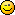Nisi Quam Primum, Nequequam

Offline

#866 2007-11-14 21:56:46

Evalesco
Member
Registered: 2007-11-14
Posts: 1

Re: 0.9999....(recurring) = 1?

Hi guys, well this is a really long thread. I can see why, I remember I had a really hard time convincing my friends of this fact as its quite hard to imagine an infinitely long representation for a number which can be represented using one digit!!
I read the first and last page and I think I might just jump in and like Khushboo, play around with an infinite sum.

Now the proof's I've seen using the geometric series look perfectly valid and concise to me but here is another way which is longer but is a little more transparent so in my opinion it will hopefully provide some insight to those of you who are of a curious nature.

We will try to give a formal proof for the following sketch proof:
10*0.99... = 9.99...
so 10*0.99... - 0.99.. = 9.99.. - 0.99..
so 9*0.99.. = 9
so 0.99.. = 1

It is aimed at those of you who have studied sums and limits.
(Sorry in advanced about the normal text, I am new to the site and couldn't find how to add latex code lol)

let an=9/(10^1) + 9/(10^2) + 9/(10^3) + ...... + 9/(10^n)    (i)

Note that the above is just stating that an = 0.999.... where there is n 9's after the 0.
So an=0.9999... as n approaches infinity.
So lim(an) = 0.99999..... where lim(an) is the value of the sum an as n approaches infinity.

10an = (9*10)/(10^1) + (9*10)/(10^2) + (9*10)/(10^3) + ..... + (9*10)/(10^n)
So 10an = 9/(10^0) + 9/(10^1) + 9/(10^2) + ..... + 9/(10^(n-1))     (ii)

Now we subtract equation (i) from equation (ii)

10an - an = [9/(10^0) + 9/(10^1) + 9/(10^2) + ..... + 9/(10^(n-1))] - [9/(10^1) + 9/(10^2) + 9/(10^3) + ...... + 9/(10^n) ]

so 9an = 9/(10^0) + 9/(10^1) + 9/(10^2) + ..... + 9/(10^(n-1)) - 9/(10^1) - 9/(10^2) - 9/(10^3) - ...... - 9/(10^n)

so 9an = 9/(10^0) + [9/(10^1) - 9/(10^1)] + [9/(10^2) - 9/(10^2)] + ..... + [9/(10^(n-1) - 9/(10^(n-1)] - 9/(10^n)

so 9an = 9/(10^0) +  +  + ..... +  - 9/(10^n)

so 9an = 9/1 - 9/(10^n)

so an = 1 - 1/(10^n)

Now we just find the limit as n approaches infinity
lim(an) = lim(1) + lim(1/(10^n))
so lim(an) = 1 - 0
so lim(an) = 1
but remember lim(an) = 0.9999....
So 0.9999.... = 1

Cool, I hope thats all correct, I know its not the most elegant way of doing it but my aim was just to provide a little insight.

If you want to give it a try, You can use a similar technique to show 0.33... = 1/3.

Offline

#867 2007-12-06 09:21:22

222kitti_kat
Member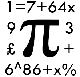Registered: 2007-12-06
Posts: 7

Re: 0.9999....(recurring) = 1?

I spent most of my maths lesson last week proving this to my friend, using the 0.333... = 1/3 and 3/3 = 0.9999999... way and eventually got her to believe me.
I like the other proofs too though, and I haven't seen most of them before....Katie...

Offline

#868 2007-12-11 16:57:47

Kargoneth
Member
Registered: 2007-08-11
Posts: 33

Re: 0.9999....(recurring) = 1?

No doubt someone has already posted this, but since I don't feel like reading through 35 pages of posts, I'll just type this anyways.

Given a single-digit integer, we can place the integer over the integer 9. When converted to decimal, the result is 0.X, where X is the single-digit integer, repeated indefinitely.

Examples:

1/9 = 0.11111111...

4/9 = 0.44444444...

9/9 = 0.99999999...

From the knowledge that any non-zero normal number divided by itself equals one, we can say that 9/9 = 1. Therefore 0.9999999999... = 1

Offline

#869 2007-12-23 19:01:32

John E. Franklin
MemberRegistered: 2005-08-29
Posts: 3,588

Re: 0.9999....(recurring) = 1?

I am writing without much explanation, but I do think that if
one is to conclude that point 9 repeating is in fact 1, then
one must also conclude that the reciprocal of zero is infinity.
However, most people like to say 1/0 is undefined.
End of statement.

P.S.  Okay, I'll explain better...
Remember the topic I mentioned that you could overload
a base-10 number with J's, which signify the number 10.
Then 100 could be written 9J since 10*9 + 10 is 100.
Now 1000 could be written 99J since 100*9 + 10*9 + 10 is 1000.
Similarly, 1.000 = 0.99J = 0.9999J = 0.99999999J = 0.999999999999J
This under our belt, we realize that J - 9 = 1.
So .99999J - .999999 = .000001
Now, since point 9 repeating forever to infinity could still
have a J at infinity, whatever infinity is, assuming there
is such an idea as infinity (I just remembered that
Ricky said a "set" could not go out to infinity, and then switch
from a 9 to a J for some reason, but just assume at
infinity, you could switch to a J), then we will for this
exercise assume that a J digit is placed at infinity.
If you can buy this interesting notion, then you can
compare point 9 repeating to this J terminated number.
By subtracting these two numbers, we hope to achieve zero in
order to prove that one equals point 9 repeating.
Remember that in base-10, each digit has a place value
that is 10 times less in value as you go to the right.
The place value of this digit is perhaps (1/10)^infinity, and
hopefully the difference between the  .9999... and .999...J is
therefore infact zero.  I am not sure if it is zero, but maybe it is,
and then .9999.... would be 1.
(J - 9)(1/10)^infinity = 0 is the proposition
Which may boil down to 1/infinity = 0
And hence 1/0 = infinity, also.
QED. (espiegle)

Last edited by John E. Franklin (2007-12-23 19:24:34)

igloo myrtilles fourmis

Offline

#870 2007-12-23 20:20:36

mikau
Member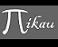Registered: 2005-08-22
Posts: 1,504

Re: 0.9999....(recurring) = 1?

I was just thinking about this the other day. It really seems like the problem is not everyone here agrees that 0.999.... is equal to its limit. But also consider sqrt(2), its decimal representation can never be fully written out, just like 0.999..'s decimal value cannot be entirely written. any decimal number with a finite number of digits will always be less than or greater than sqrt(2), but still the actual value when squared, is 2. This being the case, so what if no amount of digits makes the decimal representation of 0.999 equal to 1? are you defining its value by what the decimal digits represent? or the decimal digits themselves?

A logarithm is just a misspelled algorithm.

Offline

#871 2007-12-25 21:33:11

George,Y
Member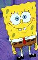Registered: 2006-03-12
Posts: 1,379

Re: 0.9999....(recurring) = 1?

John E. Franklin wrote:

I am writing without much explanation, but I do think that if
one is to conclude that point 9 repeating is in fact 1, then
one must also conclude that the reciprocal of zero is infinity.
However, most people like to say 1/0 is undefined.
End of statement.

P.S.  Okay, I'll explain better...
Remember the topic I mentioned that you could overload
a base-10 number with J's, which signify the number 10.
Then 100 could be written 9J since 10*9 + 10 is 100.
Now 1000 could be written 99J since 100*9 + 10*9 + 10 is 1000.
Similarly, 1.000 = 0.99J = 0.9999J = 0.99999999J = 0.999999999999J
This under our belt, we realize that J - 9 = 1.
So .99999J - .999999 = .000001
Now, since point 9 repeating forever to infinity could still
have a J at infinity, whatever infinity is, assuming there
is such an idea as infinity (I just remembered that
Ricky said a "set" could not go out to infinity, and then switch
from a 9 to a J for some reason, but just assume at
infinity, you could switch to a J), then we will for this
exercise assume that a J digit is placed at infinity.
If you can buy this interesting notion, then you can
compare point 9 repeating to this J terminated number.
By subtracting these two numbers, we hope to achieve zero in
order to prove that one equals point 9 repeating.
Remember that in base-10, each digit has a place value
that is 10 times less in value as you go to the right.
The place value of this digit is perhaps (1/10)^infinity, and
hopefully the difference between the  .9999... and .999...J is
therefore infact zero.  I am not sure if it is zero, but maybe it is,
and then .9999.... would be 1.
(J - 9)(1/10)^infinity = 0 is the proposition
Which may boil down to 1/infinity = 0
And hence 1/0 = infinity, also.
QED. (espiegle)

Yes, that's the tricky part to find "the last 9", John!

You are so smart!

I originally decided to reserve this part a little bit before publishing it.

Now that I don't know where to publish it yet, why not just sharing?

X'(y-Xβ)=0

Offline

#872 2007-12-25 23:11:52

George,Y
MemberRegistered: 2006-03-12
Posts: 1,379

Re: 0.9999....(recurring) = 1?

Well, traditional defense against inquiry on a "last 9" is always like this:

You cannot find a last 9, can you? Since infinity is forever, you cannot get to that (count to that).

That's a false proof though, as I refuted before, because the concept of infinity is already created unable to be reached by count.

The disproof is unsolid, but what about the proof?

My simple technique, in solid logic, but only to strengthen the intuition of 10*.999 marking the last 9:

How many 9's does .999... have?

Well, a tough question?
But first, no matter how many, the amount is neither finite nor changing.
(The precise version is that
it is not finite and stable.
it is not finite but growing.
it can be infinite but growing, but too weird to be accepted
it can then only be infinite and stable)

Now let's see the amount of 9's here
.999...
0.1*.999...
=.0999...
Notice the amount of 9's still remains the same here
.0999...+.9
=.999...#
Look! Look! Look!
We have just added in one 9 to the so-called stable infinite amount of 9's, now no matter how many 9's the original .999 has, the new .999..., marked as .999...# has Just 1 More 9 than that!

Not accepting it? Refuting it? Anyone claiming just being divided by 10 get  .999... lose one 9? If no one. Continue:

Let's just compare
the original
0.999...
and the new
0.999...#
with just one 9 more

Discompose the two from the left,
we discover that they follow the same structure
9*10[sup]-1[/sup]+9*10[sup]-2[/sup]+...
And if sorted by meaning,
the added 9 for the latter is plays just the same role as the first 9 in the former, so in this way both first 9's are the same. They are so identical that we don't say the latter one has one more 9 on the first digit or the former one has one less 9 on the first digit. (Or you get 18*10[sup]-1[/sup] or 0*10[sup]-1[/sup]

Now where is the one more 9 on .999...#?
Well, taking another angle helps:
Where is the one 9 missing in .999 compared to .999...#?

It cannot be missing on a digit before a 9 both have:
...  9...   (.999...)
...99...   (.999...#)

Note if it was lacked, it could only mean 0 at that decimal and would contradict no 0 after dot in .999. (making something like .0999 or .999...09...)

And typing a space doesn't help because the 9 before the typed space stands for the same thing as the former 9 in .999...# thus .999 doesn't lack this 9. So we just wipe out the space for simplicity.

Let me just go back to the 9 in the words "before a 9 both have".  Can we just simplify this to "before a digit which is filled by 9 in both case"? And the missing 9 in .999... is just a zero at some digit where a 9 is present in .999...#, allowing both have the same amount of digits.

Let's find the 0 and 9 combination, by the clue that there is no 9 and 9 combination after this.

The 9 in 0 and 9 combination is the last 9 in .999...#. The only 9 the .999... lacks from .999...# is the last 9 in .999...#!

The last 9 in .999...# is found! So does the last 9 in .999...! (The 9 the same to the 9 before the last 9 in .999...#)

Wait a minute! How can you say a last 9?
From beginning, I have proven the dissertation
there is no 9 and 9 combination after 0 and 9 combination.
And what does no 9 and 9 combination mean? It means either the digit is both filled with 0's or there isn't such a digit at all. Either way, the 9 in 0 and 9 combination stands for a 9 in .999...# which has no 9 following it whatsoever! That's literally what the last 9 means.

But no 9 and 9 combination, can be 9 and 0 combination ( other than 0 and 0 or nothing and nothing) -OK, then what's next? nothing ? or 9 and 9 combination again. The former, who lacks one 9? the latter, the same contradictory to full 9's.

Err, why assuming 0 and 9 combination? Because 0.999... doesn't have at least one 9 0.999...# has ( for it has one 9 less). And every 9 in 0.999...#  stands for both an existed decimal and the coefficient 9 for that decimal (9*10[sup]-i[/sup]), and it's wise to assume 0.999 has no more decimals than 0.999...#, therefore a one 9 lack means a 0 coefficient at the decimal for which 0.999...# has 9 coefficient, a 0 and 9 combination.

Hence, Logically, the last 9 is located, whose existence, is of course proven.

OK, the last 9 virus, is back! What  does it stands fpr ? What does the 9's before it stands for? Delima comes!

But this time, no one can shrink off this delima by simply denying the existence of the last 9.
Because it is not only "imagined", it is proved.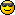Last edited by George,Y (2007-12-25 23:30:07)

X'(y-Xβ)=0

Offline

#873 2007-12-26 08:25:30

Ricky
Moderator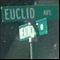Registered: 2005-12-04
Posts: 3,791

Re: 0.9999....(recurring) = 1?

We have just added in one 9 to the so-called stable infinite amount of 9's, now no matter how many 9's the original .999 has, the new .999..., marked as .999...# has Just 1 More 9 than that!

When dealing with sets of infinite size, terms such as "one more" are nonsensical.

I recommend you review your set theory, specifically dealing with mappings, the pigeon hole principle, and the continuum hypothesis.  Until you understand what "size" means when it comes to things of infinite size, you will never have a chance of understanding what is wrong with your post.

"In the real world, this would be a problem.  But in mathematics, we can just define a place where this problem doesn't exist.  So we'll go ahead and do that now..."

Offline

#874 2007-12-26 09:57:23

George,Y
MemberRegistered: 2006-03-12
Posts: 1,379

Re: 0.9999....(recurring) = 1?

terms such as "one more" are nonsensical

So you mean infinite amount is just the amount of how many integers? That's what you call mapping?

Well, that's rediculous too. Because  the infinite amount of integers is also preassumed. You count 1, then you count to 2, you count to 3, and then you assume you can count to any number. But in order to do this, you have to get a pool of infinite integers so to ensure you can have an integer as large as you want out of this pool. Otherwise how can you?

The infinite integers assumption bear the same problem. I can easily duplicate this argument by assignning one set that contains all the natural numbers plus 1, and add in the number 1 in it so it now has infinite+1 numbers due to matching.

{1, 2 , 3, 4, 5, ...}
matching by +1=
{2, 3,  4, 5, 6, ...}
above plus the set {1}=
{1, 2, 3, 4, 5, 6,...}#
Now that the subset of {1,2,3,4,5...}#, {2,3,4,5...},  is a 1-1 match to the original {1,2,3,4,5...}, the new set clearly has got 1 more element in it.
What?
You wanna argue that since you have found the pattern between the two by 1-1,2-2, 3-3, and so on, so that you are convinced that they have got all the same elements?

Is this naive inference what matching technique all about? I just see you have got 1 head, 1 chunk,  two arms, and 2 legs and therefore I conclude you and me are the same? The matching technique just naively infers equivalence by incomplete information or one sided story. It can only handle a finite amount of matching from left and never suceeds in handling the whole, even allowing that this finite amount can grow.

And my technique, on the contrary, is not only much more grounded than the matching technique but it also can spot the largest integer, discovering the largest integer problem.

What I understand is that in dealing with infinity, mathematicians always apply two meanings. They apply the real infinity subtly to demonstrate they have eliminated the residual error in case such as 1=.999..., and they prevent themselves from being spotted wrong at the last digit by forcing challengers only to think in an counting way, applying the growing infinity, which is a fake one.

I think unless you had some knowledge on the distinguishment between a real infinity and a potential one (growing one) raised by Aristotle thousands of years ago, you would never realize the mistake and the jumps from one to the other the mathematicians have made in set theory.

Last edited by George,Y (2007-12-26 10:02:52)

X'(y-Xβ)=0

Offline

#875 2007-12-26 10:10:51

Ricky
ModeratorRegistered: 2005-12-04
Posts: 3,791

Re: 0.9999....(recurring) = 1?

So you mean infinite amount is just the amount of how many integers? That's what you call mapping?

No, not at all.  I neither have the time nor the will to give you an introduction to set theory.  You are going to have to do this on your own if you wish to learn what an infinite set really is.

Because  the infinite amount of integers is also preassumed.

Zermelo-Fraenkel set theory has an infinite set axiom which says a certain type of set known as a successor set exists.  From that, the natural numbers are defined to be the smallest* such set.  Defining addition, multiplication, and exponents inductively then give us all the familiar properties of the integers.

Otherwise how can you?

Like I said, I can't give you a full introduction.  All I can do is try to help you along the way.  You are going to have to learn this stuff in depth if you really want to.

the new set clearly has got 1 more element in it.

Again, the above statement has no meaning.  Define what you mean by one more, and perhaps I'll understand what you are saying.  But you have not defined it.  It certainly has one element which is not in the other set.  But one more?  How can you conclude which set has more?

*Smallest, here, means that for any successor set, it contains an isomorphic copy of the natural numbers.

"In the real world, this would be a problem.  But in mathematics, we can just define a place where this problem doesn't exist.  So we'll go ahead and do that now..."

Offline